# Which Twin Is Older?

##### Age 16 to 18

Published 2000 Revised 2016

## Introduction

If you follow through this project, you will be led to an amazing conclusion! Suppose that one of a pair of identical twins goes on a journey into space and then returns to compare his or her (let's say her) age with that of the other twin who has remained at the same location on Earth. It turns out that after the journey, one twin will be younger than her sister! You must be asking how that could be possible, and the aim of this project is to show you how.

Let's start by playing a guessing game - we'll find out if you're right at the end! Suppose the travelling twin goes on a 12-year journey - 6 years out and 6 years back. Obviously she will be 12 years older when she gets back. What you have to do is to guess how much older the non-travelling twin will be....! I need to tell you how fast the moving twin travels - let's say four-fifths of the speed of light - although I dare say this will not help you too much with your guess! Let me suggest that you guess a whole number between 1 and 30!

Before we consider the twins, we must set up certain tools which we shall need in order to understand what is going on. We shall make a lot of use of a certain kind of graph called space-time diagram. Don't worry if you have never used graphs before - we'll try to start from the basics.
?

## Graphs

The simplest sort of graph is just a picture of what is happening on a chosen flat surface. Let's assume that you are sitting at a table or a desk, and that you have two rulers and a large piece of paper. Starting at the bottom left-hand corner, place one ruler along the bottom and one up the left-hand side, with the paper in position between them. What you have should look like this: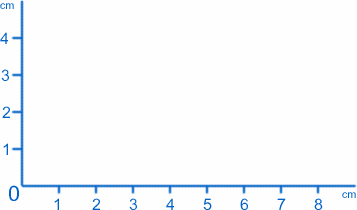We shall call the point where the two rulers meet (the zero on both scales) the origin, or O for short, and the rulers provide the two axes . Following convention, we shall call the one along the bottom the x-axis, and the one up the side the y-axis. We can label any point on the paper by its values of $x$ and $y$. For example, the origin has $x=0$, $y=0$. The point $1cm$ from the y-axis and $2cm$ from the x-axis has $x=1$, $y=2$ (I really do have these the right way round!).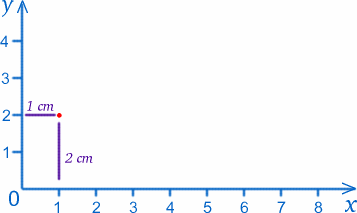Exercise Draw your own graph and mark the following points:

$x=3$, $y=0$;

$x=2$, $y=1$;

$x=3$, $y=2$;

$x=4$, $y=1$.

What shape do they form?

Now imagine some ants with very dirty feet. Suppose one walks across the paper staying always a distance of $1cm$ from the x-axis. Its path would be a line, described by the equation $y=1$ (a fancy way of saying what is said in words in the previous sentence). Another might walk always $3cm$ from the y-axis; the equation of its path would be $x=3$. A rather more original ant might walk so that its distance from both axes is always the same; it would go through $x=1$, $y=1$; $x=2$, $y=2$; etc and its path would be given by the equation $x=y$.

## Space-time diagrams

In this section, we shall draw some graphs which look very similar to those in the previous section, but their meaning will be rather different. We still have two axes, and the one labelled $x$ still represents distance in a certain direction. However the one that was labelled $y$ is now labelled $t$, which represents time, measured in seconds say. Imagine for example a ball at a fixed point $3cm$ from O; its path in space-time will be the straight line $x=3$. Now suppose the ball rolls along a straight path; then points on the graph would correspond to positions of the ball at particular times eg $x=5$, $t=4$ would correspond to the ball being $5cm$ from the origin $4$ seconds after the measurement of time began.

Exercise Plot points on the graph corresponding to the following events in the ball's history: $x=4$, $t=1$; $x=4$, $t=2$; $x=4$, $t=3$. How would you interpret this?

Now try $x=1$, $t=1$; $x=3$, $t=2$; $x=5$, $t=3$. What do you notice about these points?If the path of the ball is a straight line on the graph, it means that the ball is moving with constant speed (perhaps speed zero). In that case we can work out the speed by seeing how far the ball travels in one second - we divide the change in the x-value by the corresponding change in the t-value. So in our second example, the speed of the ball is $2cm/sec$. Do you agree?

Exercise Draw a graph representing the motion of a ball which moves backwards and forwards between two points, with constant speed (but periodically changing direction, of course).

We are now going to do something rather strange to our graph. In the theory called special relativity , which is what makes it interesting to think about about twins and space travel, we often need to plot light rays on our graphs. Now light rays travel in empty space with a constant and very large (but finite) speed; a ray of light reaching you from the sun left there about $8$ minutes ago. (People used to believe that light travelled infinitely fast so that you could see the stars at the moment you observed them but this is now known to be wrong.) To be more precise, the speed of light is about $30000000000cm/sec$! This could lead to some very strange scales on the axes of of a space-time graph, so we shall choose to measure distance in a different way; the units on the x-axis will be light-seconds, that is the distance travelled by light in one second. (If the scale for $t$ is years, the corresponding x-scale will be light-years.)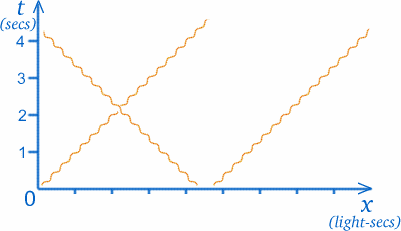The big advantage of this is that the path of a ray of light will always be at $45$ degrees to the axes. This means that a light ray through O will be at equal angles to both axes, as you see on the diagram. (Although light travels at constant speed so its path is a straight line, we represent it by a wiggly line, as shown, to distinguish light rays from paths of other objects with mass.)
?

## Measuring time

We tend to assume that when people disagree about what time it is, someone's watch is wrong, or perhaps the people are in different time zones! But it is even more complicated than that! According to special relativity, two people can be in the same place and correlate their watches, but if one is moving relative to the other, they will subsequently disagree about what time it is. Unfortunately if you team up with a friend and try to test this theory, you will be disappointed, not because the theory is wrong, but because at the speed you are likely to be able to run, even if you are a super-athlete, the effect will be too small to observe. It is only when speeds becone very large (sizeable fractions of the speed of light) that this strange phenomenon can be observed and, even then, only by using extremely accurate clocks.

To see how this could work, let us consider a "thought experiment" similar to one which Einstein suggested. (It is a "thought" experiment rather than a real one because it cannot actually be done, as you will realise.) Suppose you watch the clock on Big Ben through a very powerful telescope, as you move away from it on a very fast train which passed the clock at exactly midday (I know trains don't go right past Big Ben but let's pretend!). Now if the train could move at the speed of light, what would you see happening to the hands of the clock? They would appear to stand still, both pointing to $12$! Why? This is because the light emitted by (or reflected from) the hands at midday would be travelling away from the clock at exactly the same speed as you on the train, and light emitted at later times could not catch up with the train! Weird!

Can you see why this is a "thought experiment" (quite apart from the fact that trains don't go past Big Ben)? It is because a train could not actually move with the speed of light (which is something else that special relativity tells us - any object which weighs anything can never move as fast as light). But suppose the train moved at half the speed of light (still very fast) - you would see the clock hands move, but more slowly than those on your watch! Measurement of time depends on how you are moving!

How can this be? To understand this, let's consider a special sort of clock, a light clock . You are not likely to find one of these beside your bed waking you up in the morning. It consists of a source of light which emits signals which travel a distance D and are then reflected back to the source. The time gap or interval between each time a signal is sent and when it is received back, defines the ticks of the clock; they occur at time $2T$ apart, where \begin{equation} {T} = {D/c} \end{equation} with $c$ representing the speed of light. (Remember that speed = distance/time, so time = distance/speed.)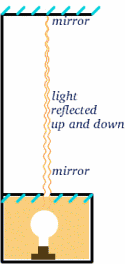Now let's suppose that a moving rocket carries such a clock - the experience of the crew will be that the ticks occur at intervals of $2T$. Now suppose that the crew of a stationary rocket observe the clock of the moving one, and compare it with their own, also ticking away at intervals of $2T$. What will they see? The diagrams below should suggest the answer. (I am sorry that my rockets look more like fish!)For the moving rocket, the light is reflected from the mirror at the half-way time between when the signal is sent and when it is reflected back. From the stationary rocket, the travel time appears to be $2T^{ \prime}$ say. We can work this out using a very important theorem (a fancy name for something that has been proved to be true in mathematics!) - that of Pythagoras.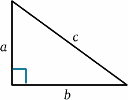\begin{equation} {a^2 + b^2} = {c^2} \quad (1) \end{equation} In the rocket diagram, we have a right-angled triangle, so \begin{equation} D^2 + v^2 T^{'2} = {c^2 T ^{\prime 2}}\quad (2) \end{equation} We now solve this for $T^{\prime}$: \begin{equation} {T^{\prime 2} (c^2 - v^2)} = {D^2}, \quad (3) \end{equation} \begin{equation} {T^{\prime 2} c^2 (1 - v^2/{c^2})} = {D^2} \quad (4), \end{equation} giving \begin{equation} {T^{\prime}} = {\frac {D} {c \sqrt{(1 - v^2/{c^2})}}}\quad (5) \end{equation} and so \begin{equation} {T^{\prime}} = {\frac {T} {\sqrt{(1 - v^2/{c^2})}}}, \quad (6) \end{equation} and the clock rates will be different (unless of course $v=0$). In fact $T^{\prime}$ is larger than $T$, so it looks to the stationary crew as though the moving clock has longer intervals between the ticks and so is going slow.

Once we accept that there is no universal definition of time which holds for everyone, we have to think what we really mean by measuring time. In some sense, we are measuring distances along our paths in space-time (think back to the space-time diagram). The twins follow different paths in space-time, so it is not so surprising that they have experienced different amounts of time.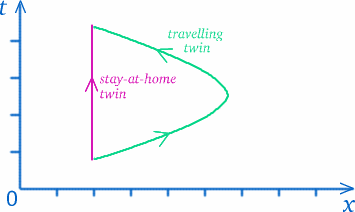But don't jump to any conclusions - things are not always what they seem...! The path of the travelling twin looks longer but does that mean she has experienced more time? To see how tricky this sort of question is, think about a situation which might be rather similar. Suppose that you live in Trumpington, on the south side of Cambridge, and want to go shopping in Tesco's on the northern extreme of the city centre (as nearly as you can, avoiding pedestrian zones), on the ring road or on the by-pass - see the diagram below.At most times of the day, you would find that the longest route - the by-pass - took the least time, followed by the ring road. Are you now convinced that the obvious answer is not always the right one??

We have seen how time is measured using light signals. Once we have an accurate clock, we can then measure distance using light or radar signals reflected from the distant object; the distance will be half the light travel time, multiplied by the speed of light.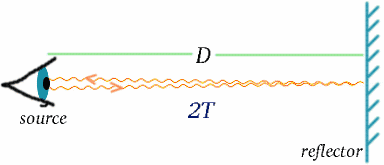\begin{equation} {D} = {cT}\quad (7) \end{equation} Like time, distance is a quantity where the result of the measurement depends on the way the observer is moving.

## K-factors

I am now going to describe an idea which should help us to do calculations of the type of effect I have been telling you about.

Suppose an astronaut B (for Ben) is in a rocket moving at speed $c/5$ away from another astronaut A (for Alf) at a space station. Once a year on March 13, Alf sends birthday greetings to Ben. Suppose that the radio message carrying this greeting in the year 2010 is measured by the space-station to travel a distance of half a light-year to reach the rocket, taking half a year to do this. The next message is sent exactly a year later. When this radio signal has travelled for half a year to where Ben the astronaut received the previous signal, the rocket has moved one-fifth of a light-year further on, so this signal has to travel for longer to catch up with the rocket; in fact, the time measured by Alf when the signal reaches the rocket is three-quarters of a year after it was sent - see the diagram below. Poor Alf concludes that the birthday greetings sent yearly will be received by Ben at intervals of one and a quarter years, according to Alf's clock.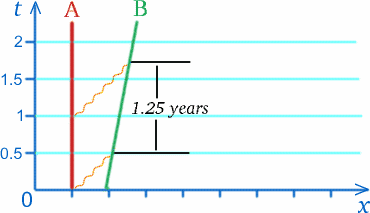This does not tell us what Ben will measure for these intervals, but it does suggest that it may well not be a year! A similar effect will happen for signals sent from Ben to Alf.

Now let's look at the general case and make this more precise. Consider two observers Alf and Ben moving away from each other at constant speeds.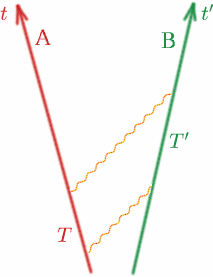Alf sends a light signal and then another at time $T$ later. Ben receives the two signals at times $T^{\prime}$ apart, according to his clock. Then we define a quantity $K$ by \begin{equation} {K} = {T^{\prime}/T}. \quad (8) \end{equation} Note that if Alf and Ben were moving with the same speed (in the same direction!), $K$ would be one. We shall see later precisely how $K$ depends on the relative speed of Alf and Ben. $K$ is sometimes called the Doppler shift factor and the effect is similar to that for sound waves - you must all know the change in sound of the siren of an ambulance or police car as it approaches and then recedes.

How can we measure $K$? The obvious way is for the observers to keep records of when the light signals are sent and received so that they can work out $T$ and $T^{\prime}$, and hence $K$, when they meet later. Another possibility would be for one to have a very powerful telescope with which to watch the clock of the other (this is really the train going past Big Ben all over again!).

We need to make some assumptions about this number $K$ in order for it to be useful to us. We assume first that when Alf and Ben are moving with constant speeds, then $K$ does not depend on when $T$ and $T^{\prime}$ are measured, nor does it depend on how big $T$ is. So for example, if Alf waits twice as long between sending light signals, $K$ will be the same. See if you can fill in the numbers $T_1^{\prime}$, $T_2^{\prime}$, $T_3^{\prime}$ in this case.
?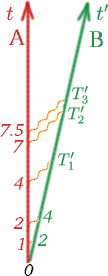(Notice that it is OK to start measuring time when the two observers are together, and they can both set their stopwatches to zero at that moment.) So in general we have
?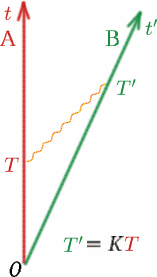The second thing we assume, which is equally important, is that the $K$ measured by Ben for light signals from Alf is the same as that measured by Alf for light signals from Ben. Why do we assume this? Imagine two identical cars back to back on a road. Car $A$ stays still and car $B$ moves off at $50km/hr$ away from $A$. Passengers looking through the back window of $A$ will see $B$ disappearing at the appropriate rate. Passengers in $B$ will see a very similar picture if they look through their back window - car $A$ will appear to be moving away at the same speed! Have you ever had that uncanny experience of sitting in a train and thinking it has just moved off, when it turns out that it was the neighbouring train moving off in the opposite direction and your train is still stationary?

This assumption makes it possible for one observer to measure $K$ by radar without any co-operation from the other. Can anyone guess how? Let me give you a hint.

Suppose as usual that Alf and Ben are moving apart with constant speed. Alf sends out two signals at an interval of $T$, Ben reflects them at interval $T^{\prime}$ and Alf receives them back again at interval $T^{\prime\prime}$. How can Alf work out $K$?
?

Answer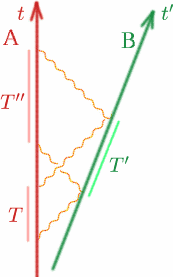\begin{equation} {T^{\prime}} = {K T} \quad (9), \end{equation} \begin{equation} {T^{\prime\prime}} = {K T^{\prime}}\quad (10) \end{equation} Therefore \begin{equation} {T^{\prime\prime}} = {K(K T)} = {K^2 T}\quad (11) \end{equation} so we have \begin{equation} {K} = {\sqrt{T^{\prime\prime}/T}}. \quad (12) \end{equation} Problem In order to perform a complicated docking manoeuvre, it is essential that two spacecraft be held at rest relative to each other. Devise a simple experiment to check that this is so.

## The relation between K and speed

We know already that if the relative speed of Alf and Ben is zero, then $K$ = $1$. What is its value for general speeds? There is a clever way of working this out, which uses the idea we have just been talking about, plus the idea of simultaneity. What does that mean? I'll explain about it in a minute.

First let's imagine our usual two observers travelling away from each other with constant speed $v$. Let's suppose that when they are together, they both set their clocks to $t = 0$. At time $T$ by his clock, Alf emits a radio signal; Ben reflects it back at time $T'$ by his clock and Alf gets it back again at time $T^{\prime\prime}$ by his clock. Let's draw a picture as usual.
?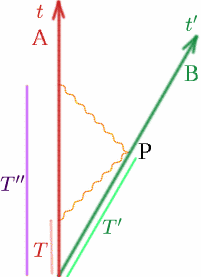Now we know that \begin{equation} {T^{\prime}} = {K T}\quad (13) \end{equation} \begin{equation} {T^{\prime\prime}} = {K T^{\prime}} = {K^2 T}. \quad (14) \end{equation} So Alf thinks that the travel time for the radio pulse is \begin{equation} {T^{\prime\prime}-T} = {K^2 T-T} = {(K^2-1)T}, \quad (15) \end{equation} and Alf works out that the distance, $D$, between himself and Ben, is half the distance travelled by the radio signal , which is half the speed times the time: \begin{equation} {D} = {\frac {1} {2} c(K^2-1)T}. \quad (16) \end{equation} Now we know that the distance between Alf and Ben is constantly changing, so we have to ask when (i.e. at what time) this is the distance between Alf and Ben. This is where we need the idea of simultaneity. The distance is clearly measured when Ben is at the point $P$, but what time does this correspond to for Alf? Well, Alf knows that the radio pulse travels the same distance out and back, so the event $Q$, which Alf judges to be at the same time as $P$, will be half way between $T$ and $T^{\prime\prime}$. (We say that $Q$ is simultaneous with $P$ for Alf - it just means at the same time.) So the time at $Q$ is \begin{equation} {T_Q} = {\frac {1} {2}(T+T'')} = {\frac {1} {2} (K^2+1)T}. \quad (17) \end{equation} Thus Alf concludes that Ben has travelled a distance $D$ in time $T_Q$, so his speed $v$ is given by \begin{equation} {v} = {\frac {D} {T_Q}} = {\frac {\frac {1} {2} c(K^2-1)T} {\frac {1} {2} (K^2+1)T}}. \quad (18) \end{equation} Therefore we have \begin{equation} {\frac {v} {c}} = {\frac {K^2-1} {K^2+1}}. \quad (19) \end{equation} We can now work out $K$ in terms of $\frac {v} {c}$: \begin{equation} {(K^2+1)\frac {v} {c}} = {K^2-1}, \quad (20) \end{equation} \begin{equation} {K^2 \frac{v}{c} + \frac{v}{c}} = {K^2-1}, \quad (21) \end{equation} \begin{equation} {1+ \frac{v}{c}} = {K^2(1- \frac{v}{c})}, \quad (22) \end{equation} Therefore \begin{equation} {K^2} = {\frac {1+ \frac{v}{c}} {1- \frac{v}{c}}}\quad (23) \end{equation} and so \begin{equation} {K} = \sqrt{{\left({\frac{1+\frac{v}{c}}{1-\frac{v}{c}}}\right)}}. \quad (24) \end{equation} Try working out some values of K:

e.g. $v = c/4$ - that gives $K = \sqrt{5/3} = 1.291$.

Now try $v = c/2, 9c/10, 99c/100$.

Now it doesn't matter whether Alf is still and Ben is moving or the other way round - $K$ is still the same. What is different is if Alf and Ben are approaching each other rather than moving apart - in that case, we take the value of $v$ to be negative, but we can use the same formula.

e.g. if Alf and Ben approach each other with relative speed $c/2$, \begin{equation} K = \sqrt{{\left({\frac{1+(-1/2)}{1-(-1/2)}}\right)}} = \sqrt{{\left({\frac{1/2}{3/2}}\right)}} = \sqrt{1/3}. \quad (25) \end{equation}. Let's work out $K$ for some typical speeds:

1) airliners approaching each other with speed $1000km/hr$;

2) galaxies in our cluster moving apart with relative speeds of $500km/s$;

3) a car approaching a policeman at $100km/hr$;

4) you walking towards your friend at $5km/hr$ Do you think any of these effects would be observable? The twin paradox - at last!

What happens? Why is it a paradox? (What does the word paradox mean anyway?)

Let's consider identical twins, Albertina and Brigitta! Albertina stay at home in London, while Brigitta, the adventurous one, goes on a space trip. She travels away from Earth for 6 years, as measured by her clock, in a very fast space ship which travels at $v = 4c/5$. She then returns at the same speed for 6 years. So Brigitta measures a time of 12 years for her trip - she is 12 years older when she gets back home to Albertina in London. But how much older is Albertina? Let's see whether your guess was right or perhaps just close!

Let's draw a space-time diagram: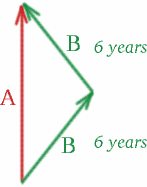On Brigitta's outward journey, the twins move apart with relative speed $v = 4c/5$, so \begin{equation} {K^2} = {\frac{1+\frac{4}{5}}{1-\frac{4}{5}}} = {9}, \quad (26) \end{equation} and we have $K=3$. On Brigitta's return journey, they approach each other with the same speed, so \begin{equation} {K^2} = {\frac{1-\frac{4}{5}}{1+\frac{4}{5}}}\quad (27) \end{equation} and $K=1/3$. All we need to do to work out the time that Albertina measures is to put in one light signal! Any suggestions where?

Suppose Albertina sends a signal at the point $S$, chosen so that it reaches Brigitta at $U$, just as she is about to turn round.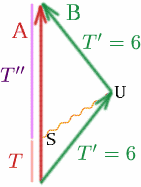Then what is $T$ if $T^{\prime}=6$ and $K=3$? We have \begin{equation} {T^{\prime}} = {K T} \quad (28) \end{equation}

so $6=3T$ and $T=2$.

Now look at what happens on Brigitta's return journey. What is $T^{\prime\prime}$? We have $T^{\prime}=6$, $K=1/3$ and \begin{equation} {T^{\prime}} = {K T^{\prime\prime}}, \quad (29) \end{equation} so $6=T^{\prime\prime}/3$ and $T^{\prime\prime}=18$. Therefore the total time measured by Albertina is $T+T^{\prime\prime}=20$ years, so she is 20 years older when they meet, whereas Brigitta is only 12 years older! So Albertina has aged by 8 years more than Brigitta!

So did you guess right????

What you can try doing on your own is some experiments with numbers. For example, you can imagine that Brigitta takes a journey of $10+10=20$ years say, and you can work out how fast she has to travel for Albertina to have aged by only one more year than she has (i.e. $21$ years has passed on Albertina's clock) when Brigitta returns. Perhaps you can work out whether it would be possible for there to be a realistic (i.e. achievable) space journey in which twins would age differently by a noticeable amount!

Now why is all this called a paradox ? Remember what paradox means? We said earlier that if two people are moving with constant speeds (and hence constant speeds relative to each other), a lot of what happens is the same whether we consider the first stationary and the second moving, or the other way round. Applying that argument to Albertina and Brigitta, why can't we regard Brigitta in her space ship as the person who sits still, and Albertina on Earth sailing away and then coming back. Put like that, it sounds rather silly, but I hope you get the idea. Then we would expect Brigitta to be older than Albertina when they finally meet up. But we have already said that Albertina is older then. How could both these statements be true? That is the paradox!

Can you see what is wrong???

The point is that Brigitta is not moving with constant speed with respect to Albertina in her entire journey; she has two long sections of journey in which she does that, but in between there is a possibly short but very important section where she decelerates, stops and then accelerates in the opposite direction. This is what makes all the difference! Acceleration is a real phenomenon, as you probably know from the rides which make you feel sick at a fair! Do you know how it could be detected with a simple piece of equipment?So what do you make of all this? It seems a bit unfair that Brigitta gets to travel and see more of the Universe but ends up younger than her stay-at-home twin sister - life does not always seem fair! But it should encourage you to travel - not necessarily in space! - and be adventurous! Reference For more discussion of the twin paradox and the techniques described here, you can try looking at the book Flat and Curved Space-Times , by G. F. R. Ellis and myself (published by Oxford University Press in 1988). You could also look at the Mr. Tompkins books which introduce the concepts of special relativity in an amusing and approachable way.

You can find reviews of the Mr Tompkins books and order them from the Cambridge University Press website, for example see The New World of Mr Tompkins : George Gamow's Classic Mr. Tompkins in Paperback